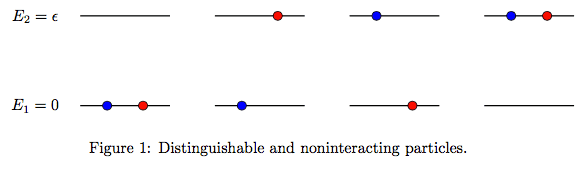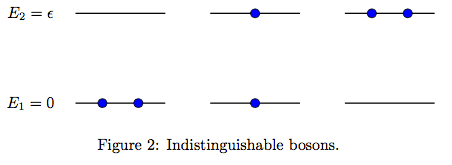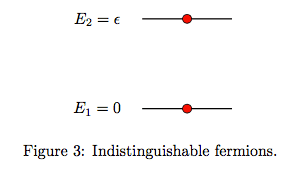# Internal Energy of a Mole of Particles each with 3 Energy Levels

Homework Statement:
A particle has three energy levels, ##E = 0##, ##\Delta##, and ##4\Delta##, where ##\Delta## is a positive constant. The lowest energy level is nondegenerate, whereas the other two are both doubly degenerate. Find the internal energy ##U## of a system of consisting of a mole of such particles.
Relevant Equations:
The canonical partition function is ##Z=\sum\limits_i g(E_i) e^{-\beta E_i}##, and the internal energy is related by ##U=-\frac{\partial}{\partial\beta} \ln Z##.
Hello, I'm doing some refreshers before going back to school. Stat mech is my shakiest and I'd appreciate some help on this problem.

I know that for a single particle, the partition function will be $$Z = 1 + 2e^{-\beta\Delta} + 2e^{-4\beta\Delta}$$ and so its internal energy is $$\frac{1}{Z} \left( 2\Delta e^{-\beta\Delta} + 8\Delta e^{-4\beta\Delta} \right).$$ My only concern is how does this extend to a mole of particles? Since each particle can be in one of 5 energy states, this means that a mole of particles will have ##5^{N_A}## energy states, with many degeneracies. In this case, is there a more explicit way to express the partition function than the general expression above?

I feel like I'm overthinking things, and it just comes down to something like tacking on an ##N_A## somewhere. If so, I'd appreciate any rough explanation why. Thank you.

In general, $$Z=z^N$$ is the partition function for a system consiting of ##N## distinguishable and noninteracting particles with single particle partition function ##z## (prove this from first principle, good exercise).

Thus, in your case where the single particle partition function is given by $$z = 1 + 2e^{-\beta\Delta} + 2e^{-4\beta\Delta}.$$ The partition function for a mole of distinguishable and noninteracting particles is therefore $$Z = \big(1 + 2e^{-\beta\Delta} + 2e^{-4\beta\Delta}\big)^{N_A}$$
and the internal energy becomes \begin{align*}U &= -\frac{\partial}{\partial\beta}\log Z \\ &= 2N_A\Delta\frac{e^{-\beta\Delta}+4e^{-4\beta\Delta}}{1+2e^{-\beta\Delta}+2e^{-4\beta\Delta}}.\end{align*}

•danyull
In general, $$Z=z^N$$ is the partition function for a system consiting of ##N## distinguishable and noninteracting particles with single particle partition function ##z## (prove this from first principle, good exercise).
Ah I remember this now! This also reminds me that I should study up on distinguishable and indistinguishable particles too. Thanks a bunch!

mjc123
Homework Helper
If the particles are indistinguishable, which is probably the case here, Z = zN/(N!). But when you take logs and then differentiate, the N! disappears.

If the particles are indistinguishable, which is probably the case here, Z = zN/(N!). But when you take logs and then differentiate, the N! disappears.
No, this is generally not true for indistinguishable particles as the following minimal example will demonstrate.

Consider a system consisting of two particles, each of which can be in one out of two possible one-particle-states with the energies ##E_1 = 0## and ##E_2 = \epsilon## respectively.

Case 1 (distinguishable and noninteracting particles)
The single-particle partition function is in this case given by \begin{align}z = 1 + e^{-\beta\epsilon}\end{align} and the total partition function is \begin{align}Z_\text{dist} &= 1 + 2e^{-\beta\epsilon} + e^{-2\beta\epsilon} \nonumber\\ &= \big(1+e^{-\beta\epsilon}\big)^2 \\ &= z^2\nonumber\end{align} as expected.

Case 2 (indistinguishable bosons)
The single-particle partition function is still given by (1). However, this time, the total partition function given by \begin{align}Z_\text{bosons} &= 1 + e^{-\beta\epsilon} + e^{-2\beta\epsilon} \nonumber\\ &\neq \frac{z^2}{2} \\ &= \frac{1}{2} + e^{-\beta\epsilon} + \frac{1}{2}e^{-2\beta\epsilon}\nonumber\end{align} as otherwise claimed.

Case 3 (indistinguishable fermions)
Likewise is the single-particle partition function also given (1). However, this is the total partition function given as \begin{align}Z_\text{fermions} &= e^{-\beta\epsilon} \nonumber\\ &\neq \frac{z^2}{2} \\ &= \frac{1}{2} + e^{-\beta\epsilon} + \frac{1}{2}e^{-2\beta\epsilon}.\nonumber\end{align}

Conclusion: Neither ##Z_\text{bosons}## nor ##Z_\text{fermions}## satisfy ##z^2/2##.

EDIT: Three pictures illustrating the above three cases.Last edited: